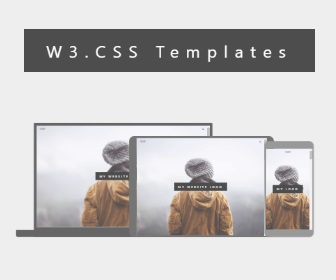## Sunday, November 5, 2023

Windows PC/Laptop-a a thlûksei le ṭ awlsam taka type theina Windows Hmar Keyboard software a hnuoia link a hin download thei a nih.

A hmang dân:

Software dang hai ang bawkin i install zova, Langauge preferences anthawk "Hmar (India) Keyboard" select el ding.

Hmet kawp ding hai:

Alt(left) + a = â

Alt(left)+Shift+a = Â

Alt(left)+e = ê

Alt(left)+Shift+e = Ê

Alt(left)+i = î

Alt(left)+Shift+i = Î

Alt(left)+t = ṭ

Alt(left)+Shift+t = Ṭ

Alt(left)+u = û

Alt(left)+Shift+u = Û

Alt(left)+q = ₹